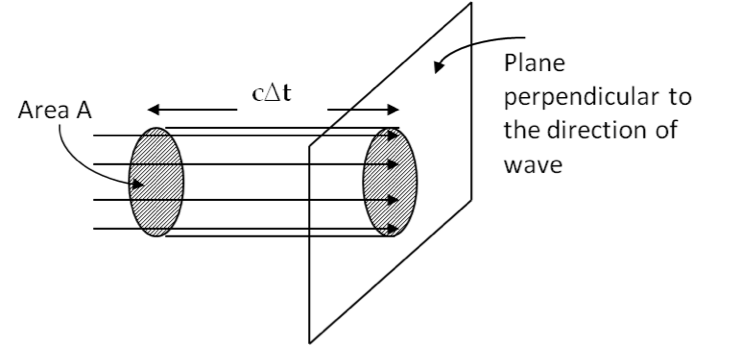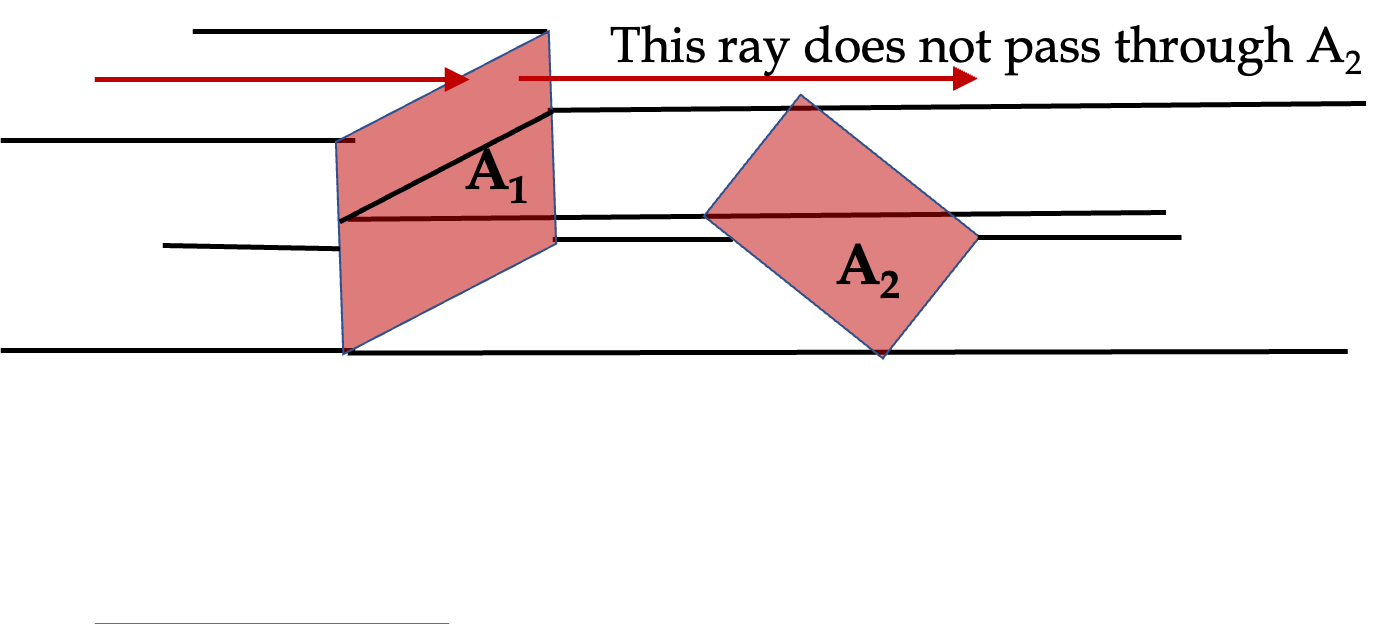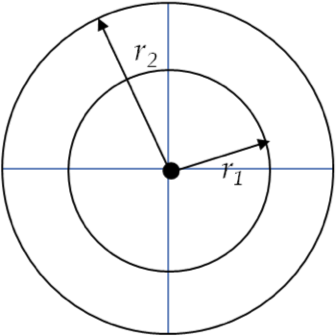## Section49.2Intensity of Light

Traveling waves transport energy from one place to another without actually moving particles. Therefore, you can think of an electromagnetic wave in terms of the flow of the electromagnetic energy.

Consider an electromagnetic wave in vacuum. The energy per unit volume contained in the oscilalting electric and magnetic fields, or energy density, to be denoted by small letter $u\text{,}$ is sum of energy in the electric field and energy in the magnetic field. From your studies of electromagnetism, you would be able to write it as

\begin{equation} u = u_E + u_B = \frac{1}{2} \epsilon_0 \vec E \cdot \vec E +\frac{1}{2 \mu_0} \vec B \cdot \vec B. \tag{49.2.1} \end{equation}

For a plane electromagnetic wave, we plug in the expressions for electric and magnetic waves given above and obtain the following for energy per unit volume.

\begin{align} u \amp = \frac{1}{2} \epsilon_0 E_0^2 \cos^2 (k x - \omega t + \phi) + \frac{1}{2 \mu_0} \left( \frac{E_0^2}{c^2} \right) \cos^2 (k x - \omega t + \phi),\notag\\ \amp = \epsilon_0 E_0^2 \cos^2 (k x - \omega t + \phi). \label{eq-EM-wave-intensity-1}\tag{49.2.2} \end{align}

Now, we look at how much energy is the wave transporting in unit time. We will use the setup given in Figure 49.2.1. Since the $u$ wave is moving at the same speed $c$ as the EM wave, the energy contained in the volume $Ac\Delta t$ will pass through area $A$ in time $\Delta t\text{.}$ That is,

\begin{equation} \text{Energy passing area } A \text{ in time } \Delta t = u \times \text{volume} = u A c\Delta t.\label{eq-EM-wave-energy-passing-through-area-in-time}\tag{49.2.3} \end{equation}Figure 49.2.1. Energy $uAc\Delta t$ contained in volume $Ac\Delta t$ will pass through an area $A$ in a plane perpendicular to the flow in time $\Delta t\text{.}$

Dividing Eq. (49.2.3) by $A\Delta t\text{,}$ will give us power flow per unit corss-sectional area. This is called power flux and denoted by $S\text{.}$ Using various equations above, we can express it as

\begin{align} S \amp = \frac{ u A c\Delta t }{A \Delta t} = u c, \tag{49.2.4}\\ \amp = c \epsilon_0 E_0^2 \cos^2 (k x - \omega t + \phi). \tag{49.2.5}\\ \amp = \frac{1}{2}c \epsilon_0 E_0^2 \left[ 1 + \cos (2k x - 2\omega t + 2\phi)\right]. \label{eq-power-flux-in-form-twice-the-angle}\tag{49.2.6} \end{align}

Since the frequency of the visible light is very high (of the order of $10^{14}$ cycles per second) the power flux will be an extremely rapidly varying quantity. Our eyes as well as most light energy measuring instruments cannot respond this fast and only an average energy over many cycles is observed. The quantity obtained by time-averaging the power flux is called the intensity or irradiance and it is normally denoted by $I\text{.}$

\begin{equation} \text{Intensity,}\ \ I = \langle S \rangle_{\text{time-average}}\label{eq-intensity-of-light-relating-to-power-flux}\tag{49.2.7} \end{equation}

If we know how much power is flowing on average over, $P_\text{av}$ some area of cross-section A, then this definition gives us intensity immediately.

\begin{equation} I = \frac{P_\text{av}}{A}.\tag{49.2.8} \end{equation}

Eq. (49.2.7) can also be used to relate intensity to electric field and magnetic field. Note that time-averaging of the expression for the power flux given in Eq. (49.2.6) is easily performed by noting that it has a constant part and a part that fluctuates between $-1$ and $1\text{.}$ The avergage of the fluctuating part over any integral multiple of cycles will be zero. Therefore, time average over long times will give a zero for the fluctuating part.

\begin{equation*} \langle \cos (at) \rangle_{\text{time-average}} = \lim_{T\rightarrow \infty} \frac{1}{T}\int_0^T \cos (at)\; dt = 0. \end{equation*}

Hence, it is easy to see that intensity of light of light wave will be given by

\begin{equation} I = \frac{1}{2} c \epsilon_0 E_0^2.\label{eqn-intensity-of-light-in-vacuum}\tag{49.2.9} \end{equation}

Since amplituds of electric and magnetic waves are related by speed of light, we can write intensity also as

\begin{equation} I = \frac{1}{2} c \epsilon_0 E_0^2 = \frac{c}{2\mu_0} B_0^2.\tag{49.2.10} \end{equation}

If the wave is not in vacuum but in some other linear medium such as glass or air we will need to replace the speed $c$ by the speed $v$ in the medium, the permittivity $\epsilon_0$ by $\epsilon\text{,}$ and the permeability $\mu_0$ by $\mu\text{.}$

\begin{equation} I = \frac{1}{2} v \epsilon E_0^2 = \frac{v}{2\mu } B_0^2.\ \ \ \text{(Linear medium)}\tag{49.2.11} \end{equation}

### Subsection49.2.1Power Flow Through Slanted Surface

In Figure 49.2.2, notice that some of the rays passign through $A_1$ will not pass through area $A_2$ even though the two surfaces are equal in value. This is due to mismatch of the perpendicular to the area direction and the direction of the wave. It is easy to incorporate this if we use vector notation for the area.Figure 49.2.2. Energy flow through two equal surfaces. Surface $A_1$ is perpendicular to the wave and $A_2$) is slanted. Less power flows through $A_2$ than $A_1\text{.}$

Introduce a power flux vector $\vec S\text{,}$ also called the Poynting vector, by the following defining equation.

\begin{equation} \vec S = \frac{1}{\mu_0} \vec E \times \vec B.\tag{49.2.12} \end{equation}

The vector product of $\vec E$ and $\vec B$ makes it evident that the Poynting vector $\vec S$ points in the direction of the propagation of the electromagnetic wave. Now, that takes care of the direction of the energy wave. Now, to take care of the orientation of the area, we introduce an area vector of a plane by its magnitude equal to the area and its direction perpendicular to the surface. Let $A$ be the magnitude of the area and $\hat n$ a unit vector in the perpendicular direction. Then, define area vector as

\begin{equation} \vec A = A\;\hat n.\tag{49.2.13} \end{equation}

Actually, there are two normal directions to a flat area. Here, we choose it arbitrarily. Then energy crossing an area vector $\vec A$ per unit time will be

\begin{equation} \vec S \cdot \vec A.\tag{49.2.14} \end{equation}

If surface is not flat but some other surface, we can divide the surface into smaller patches and define area vectors in each “flat” patch. Then on each patch, $\Delta \vec A\text{,}$ we will get

\begin{equation} \vec S \cdot \Delta \vec A,\tag{49.2.15} \end{equation}

and we must sum over all patches to obtain the total.

\begin{equation} \text{total power} = \sum_i \left( \vec S \cdot \Delta \vec A \right)_i.\tag{49.2.16} \end{equation}

To cover an arbitrary surface with flat patches, we will need infintely many patches, each of infinitesimal area. This gives the following general formula for power crossing any arbitrary surface.

\begin{equation} \text{Power crossing any surface}\ = \iint\vec S \cdot d\vec A.\tag{49.2.17} \end{equation}

### Subsection49.2.2Intensity of an Isotropic Source

Consider a point source that emits light in a spherical symmetric way. The conservation of energy can be used to show that intensity must drop off from the source as inverse square of distance.

Figure 49.2.3 shows a source of light, such as sun, at the center and two spherical surfaces in space at radii $r_1$ and $r_2\text{.}$ Let the intensities at $r_1$ and $r_2$ be denoted by $I_1$ and $I_2$ respectively.

Since energy passing the spherical surface with radius $r_1$ in an interval $\Delta t$ must equal the energy passing through the surface with radius $r_2$ in the same interval of time. Multiplying intensity with area and time gives energy. Therefore

\begin{equation*} 4\pi r_1^2 I_1 \Delta t = 4\pi r_2^2 I_2 \Delta t. \end{equation*}

Therefore,

\begin{equation*} \frac{I_2}{I_1} = \frac{ r_1^2}{r_2^2 }. \end{equation*}

This shows that the intensity of an isotropic source drops off as inverse square.Figure 49.2.3. Source is at the center and light wave is spreading out isotropically in all directions. Two circles correspond to two spherical surfaces in space.

A He-Ne laser delivers $3\text{ mW}$ of power over a $0.5 \text{cm}^2$ area.

(a) What is the intensity of light coming out of the laser?

(b) What are the amplitudes of electric and magnetic fields?

Hint

(a) Use definition of intensity. (b) Use intensity and electric field amplitude relation first.

(a) $60\text{ W/m}^2\text{,}$ (b) $213\ \textrm{N/C}\text{,}$ $7.09 \times 10^{-7}\ \textrm{T}$

Solution 1 (a)

(a) Intensity of light will be just power per unit area, since power as given is already time-averaged.

\begin{align*} I \amp = \frac{P_\text{av}}{A} = \frac{3\text{mW}}{0.5 \text{cm}^2}\\ \amp = \frac{3\times 10^{-3}\text{W}}{0.5\times 10^{-4} \text{m}^2} = 60\text{ W/m}^2. \end{align*}
Solution 2 (b)

(b) From $I = \frac{1}{2}c\epsilon_0E_0^2\text{,}$ we get

\begin{align*} |E_0| \amp = \sqrt{ \frac{2 I}{c\epsilon_0} } \\ \amp = \sqrt{ \frac{2 \times 60}{3\times 10^8 \times 8.85\times 10^{-12} } } = 213\ \textrm{N/C} \end{align*}

The magnetic and electric fields in an electromagnetic wave are related as follows.

\begin{align*} B_0 \amp = \frac{E_0}{c} \\ \amp = \frac{213\ \textrm{N/C}}{3\times 10^8\ \textrm{m/s}} = 7.09 \times 10^{-7}\ \textrm{T}. \end{align*}

A spherical wave from a point source at the origin has an electric field that drops with the radial distance $r$ from the origin as follows.

\begin{equation*} \vec E = \hat u_{\phi} \frac{V_0}{r}\ \cos(k r - 2\pi f t), \end{equation*}

where $V_0 = 10\text{ V}\text{,}$ $f = 2 \times 10^{14}\text{ Hz}\text{,}$ and $k$ is the wave number. Assume speed of light to be equal to the speed of light in vacuum.

(a) Find the wavelength of the electromagnetic wave.

(b) What is the intensity of light at $r = 1\text{ cm}\text{?}$

(c) What is the intensity of light at $r = 2\text{ cm}\text{?}$

Hint

(a) Use $\lambda f = c\text{.}$ (b) Use $I= \frac{1}{2}c\epsilon_0 E_0^2\text{.}$ (c) Recall for spherically symmetric waves $I\sim 1/r^2\text{.}$

(a) $1.5\times 10^{-6}\text{ m}\text{,}$ (b) $1330\text{ W/m}^2\text{,}$ (c) $333\text{ W/m}^2\text{.}$

Solution 1 (a)

(a) Using $\lambda f = c\text{,}$ we get

\begin{equation*} \lambda = \frac{c}{f} = \frac{3\times 10^{8}\text{ m/s} }{2 \times 10^{14}\text{ Hz}} = 1.5\times 10^{-6}\text{ m}. \end{equation*}
Solution 2 (b)

(b) Using $I= \frac{1}{2}c\epsilon_0 E_0^2$ with $E_0 = V_0/r\text{.}$

\begin{align*} I \amp = \frac{1}{2}\times 3\times 10^8 \times 8.85\times 10^{-12} \times \left( \frac{10}{0.01}\right)^2,\\ \amp = 1330\text{ W/m}^2. \end{align*}
Solution 3 (c)

(c) For spherical waves, $I$ will drop off as square of distance. Therefore, we can get intensity at $2\text{ cm}$ by simply using the value in (b).

\begin{equation*} I = \left( \frac{ 1\text{ cm}}{ 2\text{ cm}}\right)^2\ I_\text{at 1 cm}. = \frac{1330 333\text{ W/m}^2}{4} = 333\text{ W/m}^2. \end{equation*}